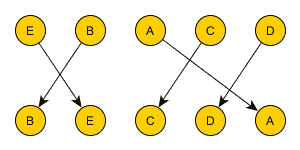# ML Wiki

## Inversion Count

Sequence inversion

• In a sequence $\pi = \langle a_0, ..., a_t \rangle$ or elements $A = \{ a_i \}$
• a pair $(a_i, a_j)$ is an inversion if $i < j \land a_i > a_j$
• the number of such inversions is the inversion number of sequence $\pi$
• this is a measure of "sortedness" of sequence $\pi$

Two ranked vectors

• An inversion in two rankings $r_1, r_2$ of the same variable $X$ is
• a pair $(x_i, x_j) \ | \ r_1(x_i) < r_1(x_j) \land r_2(x_i) > r_2(x_j)$
• it's called a pair-wise disagreement between two ranking lists

### Graphical Counting

we can represent two rankings as a Bipartite Graph $G = \langle N, S, E \rangle$

• $N = r_1(X)$ and $E = r_2(X)$ being two disjoint set of nodes
• $X$ is some variable, and $r_1$ and $r_2$ are different rankings of this variable
• $E$ is set of edges $E = \Big\{ \big(r_1(x), r_2(x) \big) \Big\}$ i.e. corresponding elements of $X$ are connected in this graph

Counting:

• bilayer drawing of $G$ is when there are two parallel lines, edges of $N$ are drawn on one, and edges of $S$ are drawn on another
• bilayer cross count is a pairwise intersections edges of $N$ and $S$
• bilayer cross count corresponds to the number of inversions when $N$ and $S$ are ranking vectors

Example:

• $X = \{ A, B, C, D, E \}$
• two ranking $r_1 = \langle E, B, A, C, D \rangle$ and $r_2 = \langle B, E, C, D, A \rangle$
• draw this is a bipartite graph and count the number of intersections
•• so there are 3 inversions in these two rankings

### Algorithms

A modification of Merge Sort can compute the # of inversions in $O(|N| \log |N|)$